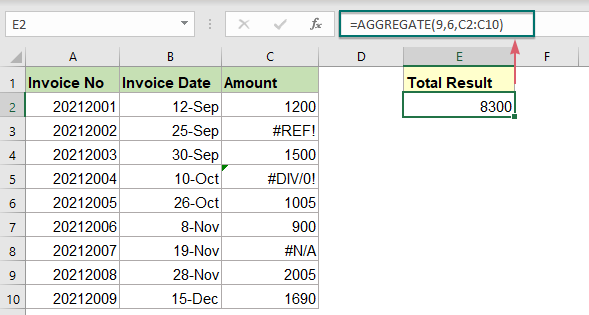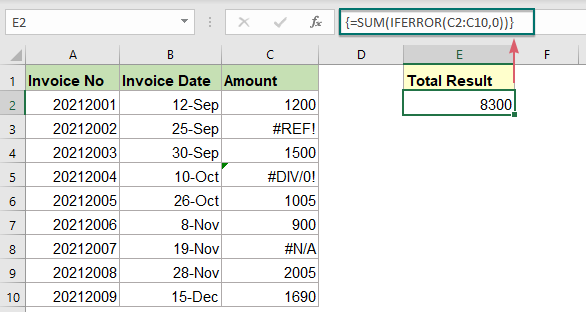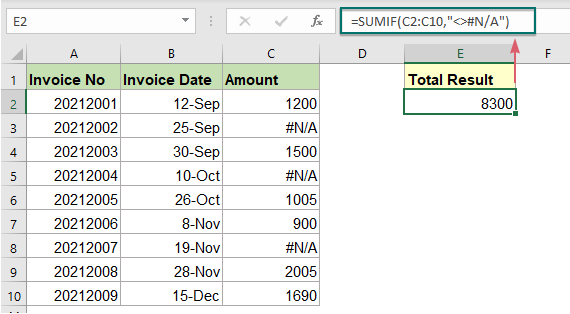## Sum all number cells ignoring errors in Excel

When summing a range of numbers which contain some error values, the normal SUM function will not work correctly. To sum only numbers and skip the error values, the AGGREGATE function or the SUM along with the IFERROR functions can do you a favor.#### Sum numbers ignoring various errors with AGGREGATE function

In Excel, the AGGREGATE function can help to sum a range of cells and ignore all kinds of errors, the generic syntax is:

=AGGREGATE(9,6,range)
• 9: This is the argument - function-num within the AGGREGATE, it indicates Sum function;
• 6: This is another argument – options within the AGGREGATE, it used to ignore error values;
• range: The range of cells to be summed.

Please apply the below formula into a blank cell, and then press Enter key to get the summation:

=AGGREGATE(9,6,C2:C10)#### Sum numbers ignoring various errors with SUM and IFERROR functions

You can also combine the SUM and IFERROR functions together to create an array formula for solving this task, the generic syntax is:

{=SUM(IFERROR(range,0))}
Array formula, should press Ctrl + Shift + Enter keys together.
• range: The range of cells you want to sum ignoring the errors.

Enter the following formula into a blank cell, and then press Ctrl + Shift + Enter keys to get the correct result, see screenshot:

=SUM(IFERROR(C2:C10,0))##### Explanation of the formula:

=SUM(IFERROR(C2:C10,0))

• IFERROR(C2:C10,0): This IFERROR function will return 0s when error values are found. So, you will get the array list as this: {1200;0;1500;0;1005;900;0;2005;1690}.
• SUM(IFERROR(C2:C10,0))=SUM({1200;0;1500;0;1005;900;0;2005;1690}): The SUM function sums all numbers in the array list to get the final result: 8300.

#### Sum numbers ignoring specific error with SUMIF function

The above two methods sum range of cells ignoring all kinds of errors. If your data contains only one error type, such as #N/A error, to ignore this specific error when summing, the SUMIF function can help you. The generic syntax is:

=SUMIF(range,"<>error_type")
• range: The range of cells you want to sum;
• error_type: A type of error that you want to ignore when summing, such as #N/A, #REF!...

In a blank cell, please enter the below formula, and then press Enter key to get the result as you need:

=SUMIF(C2:C10,"<>#N/A")Note: When applying this formula, if other types of error occur in the data list, this SUMIF function will return an error result.

#### Relative function used:

• SUMIF:
• The SUMIF function can help to sum cells based on one criterion.
• IFERROR:
• The IFERROR function is used to return a custom result when a formula evaluates an error, and return a normal result when no error is occurred.
• AGGREGATE:
• The AGGREGATE function can apply different aggregate functions to a list or database with the option to ignore hidden rows and error values.

#### More articles:

• Sum Smallest Or Bottom N Values
• In Excel, it is easy for us to sum a range of cells by using the SUM function. Sometimes, you may need to sum the smallest or bottom 3, 5 or n numbers in a data range as below screenshot shown. In this case, the SUMPRODUCT together with the SMALL function can help you to solve this problem in Excel.
• Sum Smallest Or Bottom N Values Based On Criteria
• In previous tutorial, we have discussed how to sum the smallest n values in a data range. In this article, we will perform a further advanced operation – to sum the lowest n values based on one or more criteria in Excel.
• Subtotal Invoice Amounts By Age
• To sum the invoice amounts based on age as below screenshot shown may be a common task in Excel, this tutorial will show how to subtotal invoice amounts by age with a normal SUMIF function.

### The Best Office Productivity Tools

#### Kutools for Excel - Helps You To Stand Out From Crowd

 Popular Features: Find, Highlight or Identify Duplicates  |  Delete Blank Rows  |  Combine Columns or Cells without Losing Data  |  Round without Formula ... Super VLookup: Multiple Criteria  |  Multiple Value  |  Across Multi-Sheets  |  Fuzzy Lookup... Adv. Drop-down List: Easy Drop Down List  |  Dependent Drop Down List  |  Multi-select Drop Down List... Column Manager: Add a Specific Number of Columns  |  Move Columns  |  Toggle Visibility Status of Hidden Columns  |  Compare Columns to Select Same & Different Cells ... Featured Features: Grid Focus  |  Design View  |  Big Formula Bar  |  Workbook & Sheet Manager | Resource Library (Auto Text)  |  Date Picker  |  Combine Worksheets  |  Encrypt/Decrypt Cells  |  Send Emails by List  |  Super Filter  |  Special Filter (filter bold/italic/strikethrough...) ... Top 15 Toolsets:  12 Text Tools (Add Text, Remove Characters ...)  |  50+ Chart Types (Gantt Chart ...)  |  40+ Practical Formulas (Calculate age based on birthday ...)  |  19 Insertion Tools (Insert QR Code, Insert Picture from Path ...)  |  12 Conversion Tools (Numbers to Words, Currency Conversion ...)  |  7 Merge & Split Tools (Advanced Combine Rows, Split Excel Cells ...)  |  ... and more

Kutools for Excel Boasts Over 300 Features, Ensuring That What You Need is Just A Click Away...#### Office Tab - Enable Tabbed Reading and Editing in Microsoft Office (include Excel)

• One second to switch between dozens of open documents!
• Reduce hundreds of mouse clicks for you every day, say goodbye to mouse hand.
• Increases your productivity by 50% when viewing and editing multiple documents.
• Brings Efficient Tabs to Office (include Excel), Just Like Chrome, Edge and Firefox.